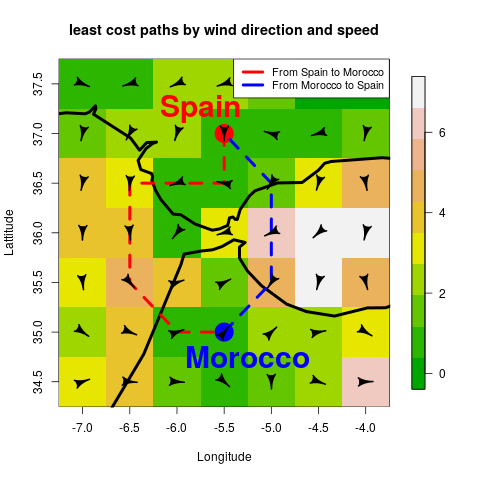# Anisotropic shortest paths across Strait of Gibraltar

#### octubre 18, 2021

First, we load the main packages we will use in this vignette. This vignette was written under the rWind version 1.1.7

# use install.packages() if some is not installed
# and you can install the latest development version using the command
# devtools::install_github("jabiologo/rWind")
library(rWind)
library(raster)
library(gdistance)

In this simple example, we introduce the most basic functionality of rWind, to get the shortest paths between two points across Strait of Gibraltar. Notice that, as wind connectivity is anisotropic (direction dependent), shortest path from A to B usually does not match with shortest path from B to A.

First, we download wind data of a selected date (e.g. 2015 February 12th).

w <- wind.dl(2015, 2, 12, 12, -7, -4, 34.5, 37.5)

Next we transform this data.frame into two raster layers, with values of wind direction and wind speed.

wind_layer <- wind2raster(w)

Then, we will use flow.dispersion function to obtain a transitionLayer object with conductance values, which will be used later to obtain the shortest paths.

Conductance<-flow.dispersion(wind_layer)

Now, we will use shortestPath function from gdistance package (van Etten 2018) to compute shortest path from our Conductance object between the two selected points.

AtoB<- shortestPath(Conductance,
c(-5.5, 37), c(-5.5, 35), output="SpatialLines")
BtoA<- shortestPath(Conductance,
c(-5.5, 35), c(-5.5, 37), output="SpatialLines")

Finally, we plot the map and we will add the shortest paths as lines and some other features.

We need some additionally packages to be installed. This can be done using the command install.packages(c("fields", "shape", "rworldmap")).

library(fields)
library(shape)
library(rworldmap)

image.plot(wind_layer$wind.speed, main="least cost paths by wind direction and speed", col=terrain.colors(10), xlab="Longitude", ylab="Lattitude", zlim=c(0,7)) lines(getMap(resolution = "low"), lwd=4) points(-5.5, 37, pch=19, cex=3.4, col="red") points(-5.5, 35, pch=19, cex=3.4, col="blue") lines(AtoB, col="red", lwd=4, lty=2) lines(BtoA, col="blue", lwd=4, lty=2) alpha <- arrowDir(w) Arrowhead(w$lon, w\$lat, angle=alpha, arr.length = 0.4, arr.type="curved")

text(-5.75, 37.25,labels="Spain", cex= 2.5, col="red", font=2)
text(-5.25, 34.75,labels="Morocco", cex= 2.5, col="blue", font=2)
legend("toprigh", legend = c("From Spain to Morocco", "From Morocco to Spain"),
lwd=4 ,lty = 1, col=c("red","blue"), cex=0.9, bg="white")